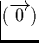Next: Addition or subtraction of Up: Auxiliary operations Previous: Negation

## Multiplication of a stream by a digit

Multiplication of a stream x by a digit a is performed by simply multiplying each digit of the stream x by the specified digit a.

If the digit is a signed binary digit, the result will be either x, the zero stream, or -x. If dyadic streams and digits are used, a dyadic digit multiplication algorithm must be used (see section A.3).

Martin Escardo
5/11/2000